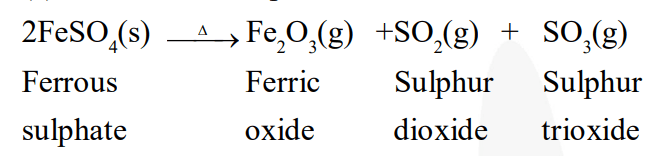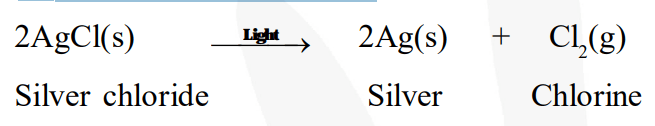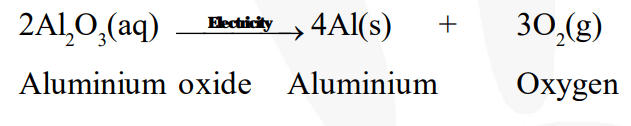# Write one equation each for decomposition reactions

Question.

Write one equation each for decomposition reactions where energy is supplied in the form of heat, light or electricity.

solution:

(a) Thermal decomposition(b) Decomposition by light(c) Decomposition by electricity